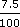Squares, roots and powers

This free course is available to start right now. Review the full course description and key learning outcomes and create an account and enrol if you want a free statement of participation.

Free course

3.2 Using scientific notation

Scientific notation can be very useful when estimating the answers to calculations involving very large and/or small decimal numbers.

Example 9

A lottery winner won £7851 000. He put the money straight into a deposit account which earns 7.5% interest per annum (i.e. each year). If he wanted to live off this interest, how much per day would it be?

The amount is £7851 000 ×÷ 365.

Estimate first to provide a check for the calculator work.

7851 000 = 7.851 × 1068 × 106= 7.5 × 10−28 × 10−2

365 = 3.65 × 1024 × 102

So the estimate becomes:

(8 × 106) × (8 × 10−2) ÷ (4 × 102).

Now separate the digits from the powers of 10 to give:

(8 × 8 ÷ 4) × (106 × 10−2 ÷ 102).

Since 8 × 8 ÷ 4 = 16 and 106 × 10−2 ÷ 102 = 106+2−2 = 102, the estimate is 16 × 102 = 1600 (£1600 a day!).

On a calculator, 7851 000 × 0.075 ÷ 365 gives £1613 rounded to the nearest pound, which is quite close to the estimate.

MU120_4M4